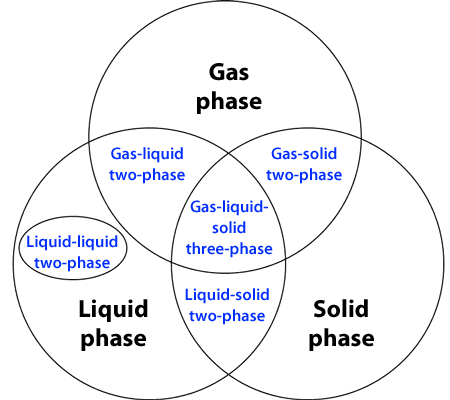Master Course for Fluid Simulation Analysis of Multi-phase Flows by Oka-san: 1. Introduction

Introduction

Yutaka Tsuji is professor emeritus in the school of Mechanical Engineering at Osaka University and is an expert in the analysis of multi-phase flows. I was first exposed to multi-phase flow analysis at one of Professor Tsuji's seminars. His seminar was full of humor which made the topic interesting to me. Multi-phase flow analysis ultimately became the focus of my studies largely due to the impact that Professor Tsuji's lecture had on me. A few years later, Grade 1 for analysis engineers in thermal fluid mechanics was added to the certification program for computational mechanics engineers sponsored by the Japan Society of Mechanical Engineers. I worked to learn the analysis skills, and passed Grade 1 for multi-phase flow analysis. And now, I am writing this column about multi-phase flow analysis.

What is multi-phase flow analysis? It is a scientific term. One way to develop an understanding of the phrase is to look at the words individually. The word “phase” in “multi-phase flow analysis” stands for the three different possible states of an object, namely the gas, liquid, or solid phases. “Multi-phase” means two or more phases are present and can be classified into four types: gas-liquid two-phase, gas-solid two-phase, solid-liquid two-phase, and gas-liquid-solid three-phase. In addition, two immiscible liquids such as water and oil are called liquid-liquid two-phase and may also be included in a multi-phase flow analysis. (See Figure 1.)Figure 1: Classification of multi-phases

A multi-phase flow analysis simulates a multiphase flow. The analysis method is computational fluid dynamics (CFD). The phrase “fluid simulation analysis” may also be used.

Example of multi-phase flow analysis

Here are some analysis examples to provide a better understanding about what a multi-phase flow analysis is. The milk crown phenomenon is a typical example of a gas-liquid two-phase flow (Figure 2). A crown shape is formed when a liquid droplet impinges against a thin liquid film. The shape of the crown is most easily captured by high-speed photography. In Figure 2, the free surface flow that is the interface between air (gas phase) and liquid (liquid phase) is analyzed.

Figure 2: Milk crown phenomenon

A cyclone separator is a good example of a gas-solid two-phase flow. The separator literally separates air and powder using the centrifugal force. This is the mechanism used in a cyclone vacuum cleaner. In Figure 3, the flow of air (gas phase) is analyzed along with the flow of the powder (solid phase) using the particle tracking method.

Figure 3: Cyclone separator

Ice-making system is a typical example of a liquid-solid two-phase flow. In Figure 4, the transparent region represents water, and white region represents ice. In this analysis, the phase change between ice (solid-phase) and water (liquid-phase) is modeled, i.e., solidification and melting.

Figure 4: Ice making

A rising oil droplet in water is an example of a liquid-liquid two-phase flow. The analysis approach depends on the number of oil droplets. In Figure 5, the free surface flow that comprises the interface between two liquid-phases is analyzed and the surface tension force is considered.

Figure 5: Oil droplet

Solidification of molten metal is an example of a gas-liquid-solid three-phase flow. Air (gas phase), molten metal (liquid phase, red region in the figure), and a solidified metal (solid phase, gray region in the figure) coexist. In Figure 6, a free surface flow and a solidification/melting model are used together to analyze the phenomenon.

Figure 6: Solidification of molten metal

These examples provide a glimpse of the kinds of multi-phase flows that can be simulated using computational analysis. However, many multi-phase flow phenomena are very complex, so not all multi-phase flows can be analyzed computationally. Even so, continued improvements in computer hardware and software capabilities are making multi-phase flow problems solvable using fluid simulation analyses.